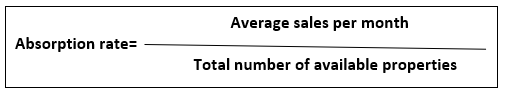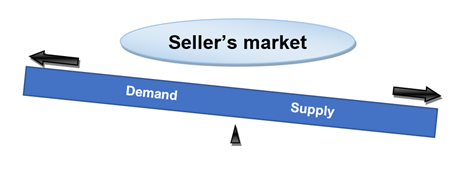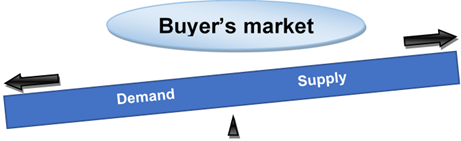# Absorption Rate

• Updated on

## What is the absorption rate?

The word absorption rate is a statistic that is often used in the real estate industry to determine the price of available residences sold in a specific area at a certain time. The absorption rate is also utilised in accounting, where it is used to calculate the overhead cost of the business organisations. The formula for calculating absorption rate is:This formula could also be used to calculate the time required to sell off all the available properties. If the absorption rate results in more than 20 percent, it is considered the seller's market. Whereas, if the absorption rate is calculated below 15 percent, it is regarded as the buyer's market.

Summary
• The absorption rate is a commonly used term in the real estate market.
• The absorption rate above 20% is considered a seller's market, while the absorption rate below 15% is regarded as the buyer's market.
• The absorption rate helps the accountants to calculate the overhead expenses.
• The absorption rate also determines the estimated time needed to sell off all the available supplies.

## What is a seller market?

A seller market can be defined as the scenario where the number of supplies is lesser than the consumers' demand. In such a situation, the number of properties is less as compared to the number of buyers. Hence, there is a high market price of properties at that time due to the increased demand. It is the ideal opportunity for a seller to make the sale at that time.A buyer market can be defined as the scenario where the supply exceeds the demand of the consumers. In such a situation, the number of properties is greater than the number of people looking forward to buying property. It is considered the ideal time for buying properties as the buyers could get it at a low price than usual.## What is the impact of absorption rate in the real estate market?

The absorption rate acts as an indication to the builders to develop new properties. An increase in the absorption rate symbolizes that there is a higher demand for houses in the real estate market, which gives assurance to the builders to build more homes. Similarly, a downfall in the absorption rate symbolizes a surplus of supply in the real estate market, and hence there is no need to build more houses.

Appraisers also use the rate of absorption to determine the correct value of a property. It is mandatory to present an appendix in some procedures to show that absorption rates were considered for appraisal calculation.

Generally, the appraisers analyze the market condition and maintain awareness about the rise and fall of the absorption rate.

## How does the rate of absorption utilised in accounting?

We have already mentioned before that the rate of absorption is also used in accounting to calculate the overhead cost of a company. Certain additional expenses are made while offering service to the clients is known as an overhead cost. It is also frequently regarded as the overhead absorption rate at times.

The overhead cost is evaluated by dividing the total budget overhead cost by the entire budget production base. It requires some adjustment at the end of the accounting as the actual cost may vary from the estimated cost.

## What is the significance of absorption rate?

Apart from being a measurement tool, the absorption rate also determines the estimated time taken to sell off all the available properties in the real estate market. The following mentioned below are the significances of absorption rate:

• Analyze the time for seller's market and buyer's market.
• During the seller's market, the seller has full authority over the price of the property.
• During the buyer's market, the buyer has the power to determine the pricing.Image source: © Feverpitched | Megapixl.com

How to calculate the rate of absorption in real estate?

The absorption rate is calculated by dividing the number of homes sold in a certain time by the total number of homes for sale. You can simply calculate the monthly rate of absorption by taking the total number of houses sold in a market and dividing it by 12.

For instance, an absorption rate of 12 can be explained as the average number of houses sold per month is 12 when the total number of homes available for sale is 100. It also denotes that it would take approximately 12 months to sell out all the properties ultimately. An absorption rate of 12 also implies a buyer's market wherein the supply is surplus, and the demand is less.

##### Top ASX Listed Companies

Definition:
We use cookies to ensure that we give you the best experience on our website. If you continue to use this site we will assume that you are happy with it. OK